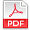## Scientific Review

Online ISSN: 2412-2599
Print ISSN: 2413-8835

Frequency: Monthly

# Archives

### Volume 1 Number 4 September 2015

#### Computation of Dielectric Constant and Loss Factor of Water and Dimethylsulphoxide from 0.1 to 13 GHzPDF

Authors: Onimisi M.Y. ; Ikyumbur J.T.
Pages: 79-85
Abstract
This study examined the computation of the dielectric constant (ε’) and dielectric loss factor (ε’’) of water and dimethylsulphoxide (DMSO)at temperature 20oc, 30oc, 40oc and 500C within the frequency range of 0.1≤f≤13GHz using Debye equations. The Debye equations were derived, and the experimental values of the static dielectric constant (ε_s), dielectric constant at high frequency (ε_∞) and the relaxation time (τ) of water and DMSO at those temperatures were substituted into the derived equations and the dielectric constant (ε’) and loss factor (ε’’) of water and DMSO were computed with aid of maple-13 and results were generated. These results revealed that the method is capable of reproducing good results forwork done using single Debye and that of the reflection cell ofdimethylsulphoxide.

#### Classification of Iris Data using Kernel Radial Basis Probabilistic Neural NetworkPDF

Authors: Mohd. Syafarudy Abu ; Lim Eng Aik
Pages: 74-78
Abstract
Radial Basis Probabilistic Neural Network (RBPNN) has a broader generalized capability that been successfully applied to multiple fields. In this paper, the Euclidean distance of each data point in RBPNN is extended by calculating its kernel-induced distance instead of the conventional sum-of squares distance. The kernel function is a generalization of the distance metric that measures the distance between two data points as the data points are mapped into a high dimensional space. During the comparing of the four constructed classification models with Kernel RBPNN, Radial Basis Function networks, RBPNN and Back-Propagation networks as proposed, results showed that, model classification on Iris Data with Kernel RBPNN display an outstanding performance in this regard.